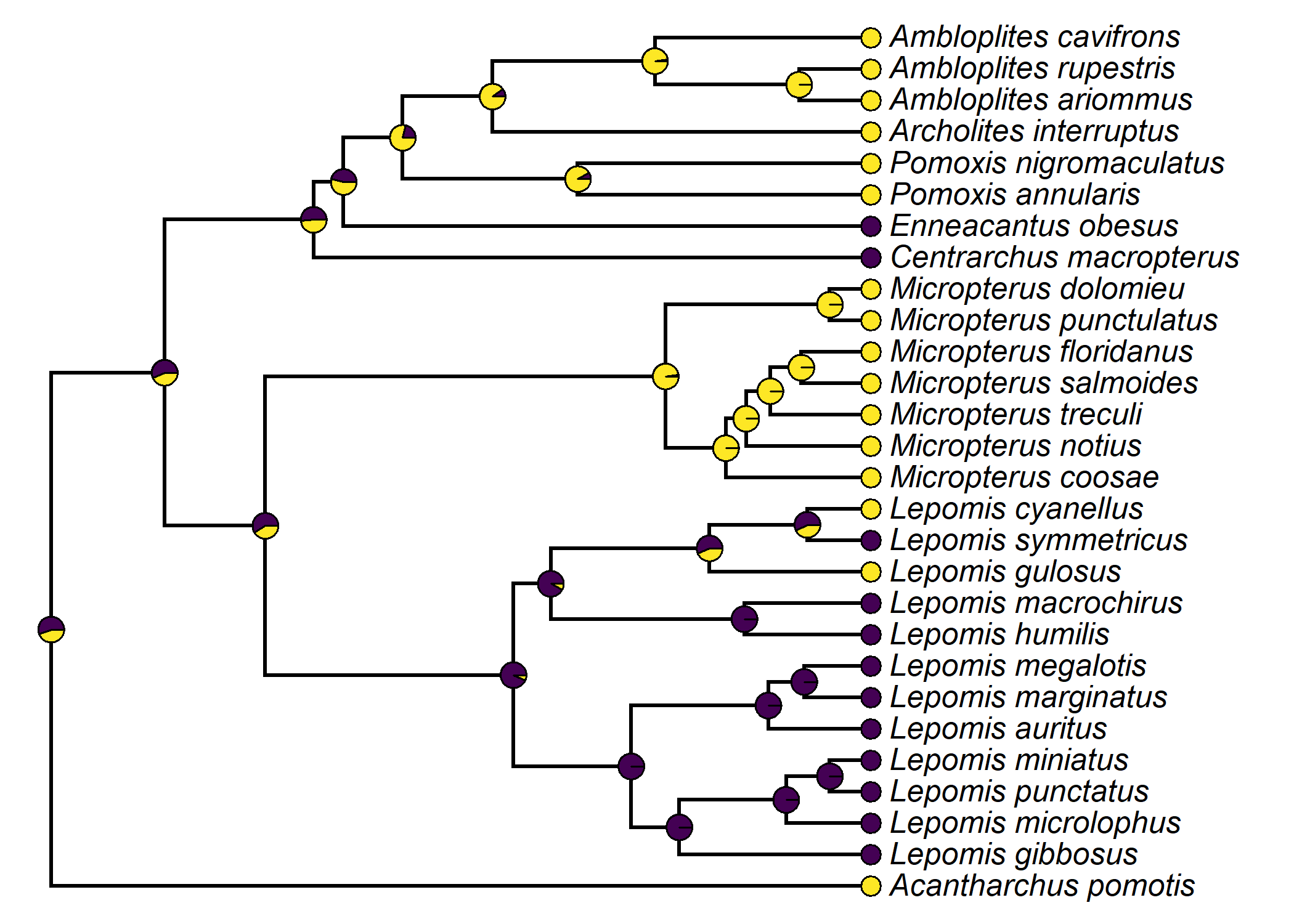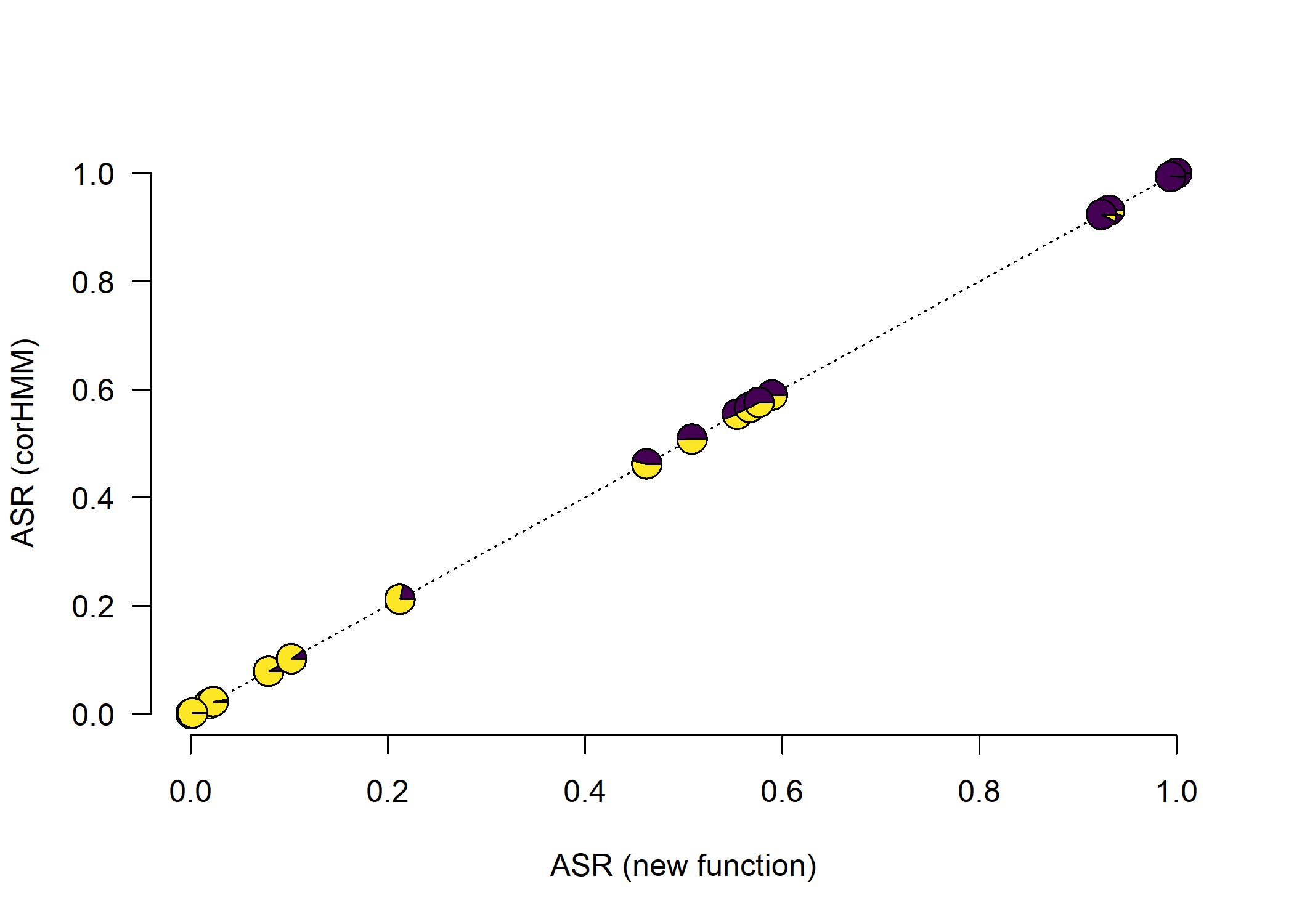## Wednesday, March 22, 2023

### Custom function for marginal ancestral state reconstruction using phytools

Yesterday, I posted a simple demonstration of Felsenstein’s pruning algorithm that can be used to compute the likelihood of a discrete character on the tree under a continuous time Markov process, for any model of transitions between conditions of that chain. This is the same algorithm that is used to compute the likelihood both by functions like `phytools::fitMk` and `geiger::fitDiscrete`, as well as by Maximum Likelihood phylogeny inference.

The pruning algorithm is a single postorder tree traversal – meaning we traverse the tree from the tips towards the root, visit every daughter node before its parent. To obtain marginal ancestral states, we need to proceed & traverse the tree again – this time in a preorder fashion, meaning from root to tips.

Let’s try it.

Firstly, here’s our pruning algorithm again. This time I’ve slightly modified this function so that it can allow the user to ask to return the matrix of “conditional” likelihoods (of each subtree) computed during pruning, instead of just the total probability of the site pattern.

``````pruning<-function(q,tree,x,model=NULL,...){
if(hasArg(return)) return<-list(...)\$return
else return<-"likelihood"
pw<-reorder(tree,"postorder")
k<-length(levels(x))
if(is.null(model)){
model<-matrix(1,k,k)
diag(model)<-0
}
if(hasArg(pi)) pi<-list(...)\$pi
else pi<-rep(1/k,k)
Q<-matrix(0,k,k)
Q[]<-c(0,q)[model+1]
diag(Q)<--rowSums(Q)
L<-rbind(to.matrix(x[pw\$tip.label],levels(x)),
matrix(0,tree\$Nnode,k,dimnames=
list(1:tree\$Nnode+Ntip(tree))))
nn<-unique(pw\$edge[,1])
for(i in 1:length(nn)){
ee<-which(pw\$edge[,1]==nn[i])
PP<-matrix(NA,length(ee),k)
for(j in 1:length(ee)){
P<-expm(Q*pw\$edge.length[ee[j]])
PP[j,]<-P%*%L[pw\$edge[ee[j],2],]
}
L[nn[i],]<-apply(PP,2,prod)
}
prob<-log(sum(pi*L[nn[i],]))
if(return=="likelihood") prob
else if(return=="conditional") L
}
``````

Now here is our marginal ancestral state reconstruction function.

``````asr<-function(q,tree,L,model=NULL){
pw<-reorder(tree,"postorder")
k<-ncol(L)
if(is.null(model)){
model<-matrix(1,k,k)
diag(model)<-0
}
Q<-matrix(0,k,k)
Q[]<-c(0,q)[model+1]
diag(Q)<--rowSums(Q)
nn<-unique(pw\$edge[,1])
for(i in length(nn):1){
ee<-which(pw\$edge[,1]==nn[i])
for(j in 1:length(ee)){
P<-expm(Q*pw\$edge.length[ee[j]])
pp<-t(L[nn[i],]/(P%*%L[pw\$edge[ee[j],2],]))
L[pw\$edge[ee[j],2],]<-(pp%*%P)*
L[pw\$edge[ee[j],2],]
}
}
anc<-L/rep(rowSums(L),k)
anc[1:Nnode(tree)+Ntip(tree),]
}
``````

We can see that (after rearranging the `edge` matrix of our tree to be in postorder direction), we traverse from the bottom to the top of this matrix. That’s the opposite of what we did in our pruning algorithm, which makes the algorithm preorder in direction.

Let’s go ahead & apply it to a case.

``````library(phytools)
library(expm)
## get our data
data(sunfish.tree)
data(sunfish.data)
fmode<-setNames(sunfish.data\$feeding.mode,
rownames(sunfish.data))
## set our model design (this is the ARD model)
model<-matrix(c(0,1,2,0),2,2,byrow=TRUE)
## estimate our model parameters
fitted<-optim(c(1,1),pruning,tree=sunfish.tree,x=fmode,
model=model,method="L-BFGS-B",lower=1e-12,
control=list(fnscale=-1))
fitted
``````
``````## \$par
##  6.087786 3.048911
##
## \$value
##  -12.86494
##
## \$counts
##       11       11
##
## \$convergence
##  0
##
## \$message
##  "CONVERGENCE: REL_REDUCTION_OF_F <= FACTR*EPSMCH"
``````
``````## now get our conditional likelihood, fixing Q
L<-pruning(fitted\$par,sunfish.tree,fmode,model=model,
return="conditional")
## finally, perform our ancestral state reconstruction
sunfish_asr<-asr(fitted\$par,sunfish.tree,L,model=model)
sunfish_asr
``````
``````##             non          pisc
## 29 0.5542907770 0.44570922300
## 30 0.5680748303 0.43192516970
## 31 0.5896468549 0.41035314510
## 32 0.9316023240 0.06839767597
## 33 0.9958981293 0.00410187067
## 34 0.9983977719 0.00160222805
## 35 0.9997184688 0.00028153118
## 36 0.9999506913 0.00004930871
## 37 0.9995868493 0.00041315072
## 38 0.9998746228 0.00012537719
## 39 0.9236549420 0.07634505797
## 40 0.9937256128 0.00627438715
## 41 0.5667238236 0.43327617637
## 42 0.5762108219 0.42378917814
## 43 0.0185497613 0.98145023868
## 44 0.0014562747 0.99854372529
## 45 0.0003363109 0.99966368906
## 46 0.0001482823 0.99985171771
## 47 0.0001806371 0.99981936286
## 48 0.0004987770 0.99950122297
## 49 0.5082533316 0.49174666843
## 50 0.4623446264 0.53765537358
## 51 0.2119419076 0.78805809236
## 52 0.0787578762 0.92124212377
## 53 0.1019695389 0.89803046110
## 54 0.0225587330 0.97744126704
## 55 0.0016423037 0.99835769630
``````
``````plotTree(sunfish.tree,ftype="i",offset=0.5)
nodelabels(pie=sunfish_asr,piecol=viridisLite::viridis(n=2),
cex=0.5)
tiplabels(pie=to.matrix(fmode[sunfish.tree\$tip.label],
levels(fmode)),piecol=viridisLite::viridis(n=2),cex=0.4)
``````That looks good, but let’s compare it to our marginal states obtained using the corHMM package by Jeremy Beaulieu et al., which is what Luke & I trusted for reliable ancestral state estimation in our recently published book.

``````dat<-data.frame(Genus_sp=names(fmode),
feeding_mode=as.numeric(fmode))
sunfish_corhmm<-corHMM::corHMM(as.phylo(sunfish.tree),dat,
node.states="marginal",rate.cat=1,
rate.mat=matrix(c(NA,1,2,NA),2,2),
root.p=c(0.5,0.5))\$states
``````
``````## State distribution in data:
## States:	1	2
## Counts:	12	16
## Beginning thorough optimization search -- performing 0 random restarts
## Finished. Inferring ancestral states using marginal reconstruction.
``````

Let’s plot a comparison. Just to make it look cool (for Twitter, etc.), I’m going to plot the marginal likelihoods as pie charts using `plotrix::floating.pie`.

``````plot(NA,xlim=c(0,1),ylim=c(0,1),xlab="ASR (new function)",
ylab="ASR (corHMM)",bty="n",las=1)
lines(c(0,1),c(0,1),lty="dotted")
nulo<-mapply(floating.pie,xpos=sunfish_asr[,1],
ypos=sunfish_asr[,1],
x=apply(sunfish_asr,1,function(x) x, simplify=FALSE),